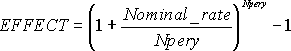## Excel Office

Excel How Tos, Tutorials, Tips & Tricks, Shortcuts

# NOMINAL function: Description, Usage, Syntax, Examples and Explanation

## What is NOMINAL function in Excel?

NOMINAL function is one of the Financial functions in Microsoft Excel that returns the nominal annual interest rate, given the effective rate and the number of compounding periods per year.

## Syntax of NOMINAL function

NOMINAL(effect_rate, npery)

The NOMINAL function syntax has the following arguments:

• Effect_rate: The effective interest rate.
• Npery: The number of compounding periods per year.

## NOMINAL formula explanation

• Npery is truncated to an integer.
• If either argument is nonnumeric, NOMINAL returns the #VALUE! error value.
• If effect_rate ≤ 0 or if npery < 1, NOMINAL returns the #NUM! error value.
• NOMINAL (effect_rate,npery) is related to EFFECT(nominal_rate,npery) through effective_rate=(1+(nominal_rate/npery))*npery -1.
• The relationship between NOMINAL and EFFECT is shown in the following equation:## Example of NOMINAL function

Steps to follow:

1. Open a new Excel worksheet.

2. Copy data in the following table below and paste it in cell A1

Note: For formulas to show results, select them, press F2 key on your keyboard and then press Enter.

You can adjust the column widths to see all the data, if need be.

 Data Description 0.053543 Effective interest rate 4 Number of compounding periods per year Formula Description Result =NOMINAL(A2,A3) Nominal interest rate with the terms above 0.05250032